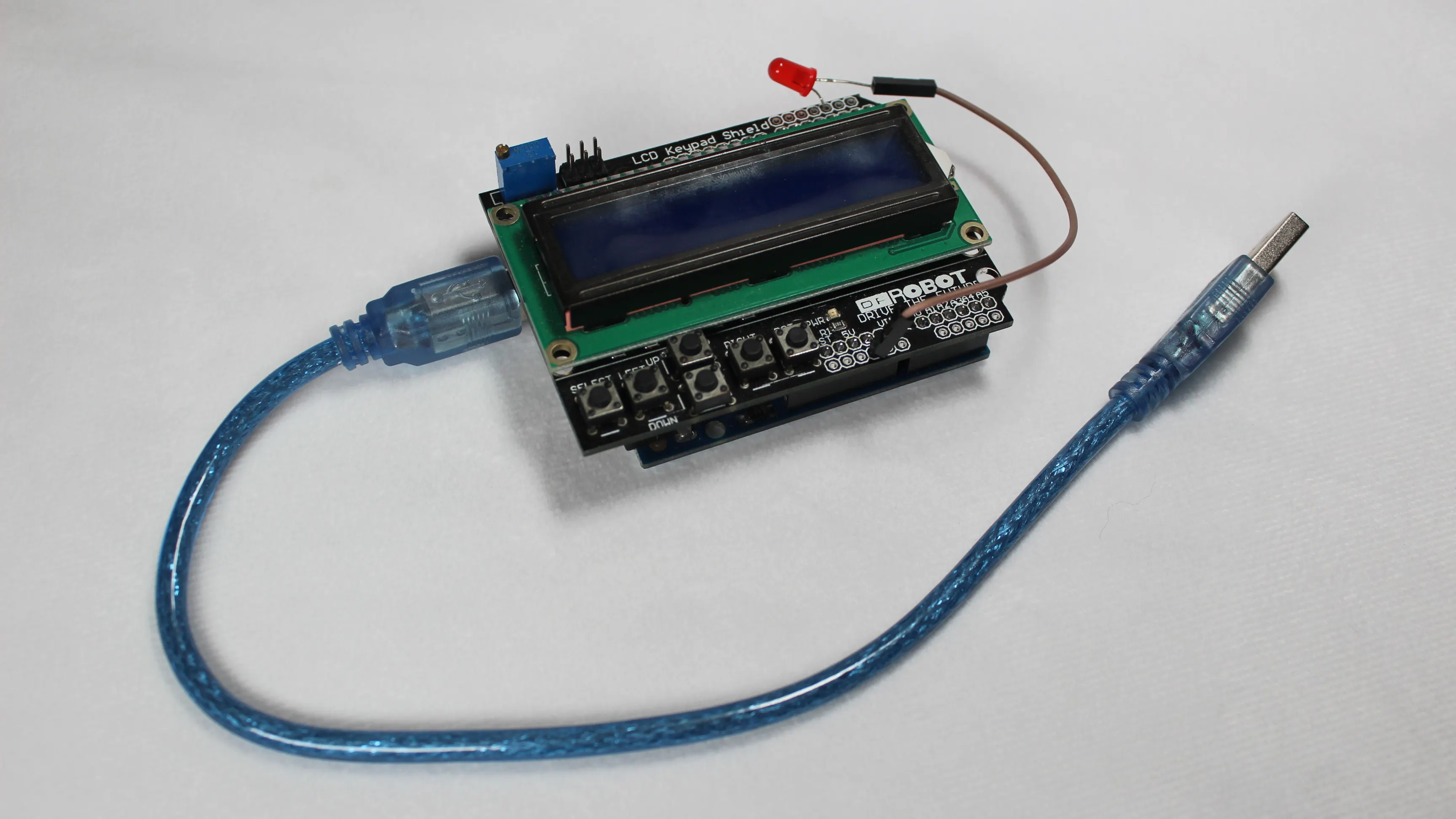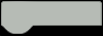# Screen Time Break for Arduino UNO

A simple and smart Arduino time keeping code that reminds computer users to take a visual break from their screen.

Things used in this project

### Hardware components

HARDWARE LIST
1 Arduino UNO
1 DFRobot 1602 LCD Keypad Shield
1 USB-A to B Cable
1 5 mm LED: Red
1 Wire, Wrapping Wire

Arduino IDE

### Hand tools and fabrication machines

Soldering iron (generic)

Solder Flux, Soldering

StoryScreen Time Break 1.0.3 for Arduino UNO and LCD Keypad Shield.

Published on Oct 4, 2022

www.alexaldridge.co

A simple and smart Arduino time keeping code that reminds computer users to take a visual break from their screen. Every 20 minutes an LED light indicates that the computer user should take a visual break from their screen. If the timer has been operating for more than 2 hours, the user is reminded to take a 20 minute break.

Press the RIGHT button for 2 seconds to start the timer. Press the LEFT button to pause the timer. Press the RIGHT button after pressing the LEFT button to resume the timer. Press the RST button to reset the timer. The timer has a limit of 24 hours and will automatically reset after 24 hours.

This software is free and open-source; anyone can redistribute it and/or modify it.

Schematics

### Screen Time Break for Arduino UNO and LCD Keypad Shield.Code

### Screen Time Break for Arduino UNO and LCD Keypad Shield

Arduino

A simple and smart Arduino time keeping code that reminds computer users to take a visual break from their screen.

CODE
``````/*
Screen Time Break 1.0.3 for Arduino UNO and LCD Keypad Shield.
Updated on Oct 7, 2022
www.alexaldridge.co

A simple and smart Arduino time keeping code that reminds computer users to take a visual break from their screen.
Every 20 minutes an LED light indicates that the computer user should take a visual break from their screen.
If the timer has been operating for more than 2 hours, the user is reminded to take a 20 minute break.

Press the RIGHT button for 2 seconds to start the timer.
Press the LEFT button to pause the timer.
Press the RIGHT button after pressing the LEFT button to resume the timer.
Press the RST button to reset the timer.
The timer has a limit of 24 hours and will automatically reset after 24 hours.

Release 1.0.3 notes:
- Fixed seconds time display error.
- Adjusted LED timing for 20 minutes.

This software is free and open-source; anyone can redistribute it and/or modify it.
*/

#include <LiquidCrystal.h>
//LCD pin to Arduino
const int pin_RS = 8;
const int pin_EN = 9;
const int pin_d4 = 4;
const int pin_d5 = 5;
const int pin_d6 = 6;
const int pin_d7 = 7;

LiquidCrystal lcd( pin_RS,  pin_EN,  pin_d4,  pin_d5,  pin_d6,  pin_d7);

int sec = 0;
int min = 0;
int hou = 0;
int bulkmin= 0, bulksec = 0, bulkhou = 0;
long start_time = 0;
boolean time_two_hour = false;

int button = 0;
int safe=0;
int pause = 0 ;

void setup(){
pinMode(2, OUTPUT);

lcd.begin(16, 2);
lcd.clear();
lcd.setCursor(2,0);
lcd.print("Screen Time");

Serial.begin(9600);

}

void loop(){

if ( button <= 60){

pause = 1;

}
if (button >=400 && button <= 600){

pause = 2;

}
if(pause == 1){

sec = sec + 1;
if(sec == 20){

digitalWrite(2, LOW);
}

if(sec == 60){
lcd.setCursor(11,1);
lcd.print(" ");
lcd.setCursor(12,1);
lcd.print(" ");
bulksec = sec;
sec = 00;
min = min + 1;

if (min == 20 || min == 40 || min == 60){

digitalWrite(2, HIGH);

}

if(min == 60){
lcd.setCursor(8,1);
lcd.print(" ");
lcd.setCursor(9,1);
lcd.print(" ");
bulkmin = min;
min = 00;
hou = hou + 1;

if((hou % 2) ==0){

digitalWrite(2, HIGH);
time_two_hour = true;
start_time = millis();

}

if(hou == 23 && bulkmin == 60){

lcd.setCursor(5,1);
lcd.print(" ");
lcd.setCursor(6,1);
lcd.print(" ");
hou = 00;
}

}
}
printTime();
Timer_Time();
}
else{

printTime();
Timer_Time();
}

delay(1000);
}

void Timer_Time()
{

if(time_two_hour == true)
{
if(start_time + 90000 <= millis())
{
digitalWrite(2, LOW);
time_two_hour = false;
}

}

}

void printTime()
{
if(hou<=9)
{ lcd.setCursor(4,1); lcd.print("0");
lcd.setCursor(5,1); lcd.print(hou); }
else
{lcd.setCursor(4,1); lcd.print(hou); }
lcd.setCursor(6,1);
lcd.print(":");
if(min<=9)
{ lcd.setCursor(7,1); lcd.print("0");
lcd.setCursor(8,1); lcd.print(min); }
else
{lcd.setCursor(7,1); lcd.print(min); }

lcd.setCursor(9,1);
lcd.print(":");
if(sec<=9)
{
lcd.setCursor(10,1);
lcd.print("0");
lcd.setCursor(11,1);
lcd.print(sec);
}
else{
lcd.setCursor(10,1);
lcd.print(sec);
}
}``````

### Screen Time Break 1.0.3

https://github.com/AlexAldridge/Arduino/blob/Screen-Time-Break/Screen_Time_Break_1.0.3.ino

The article was first published in hackster, October 4, 2022

cr: https://www.hackster.io/alexaldridge/screen-time-break-for-arduino-uno-cfb4fa

author: alexaldridge